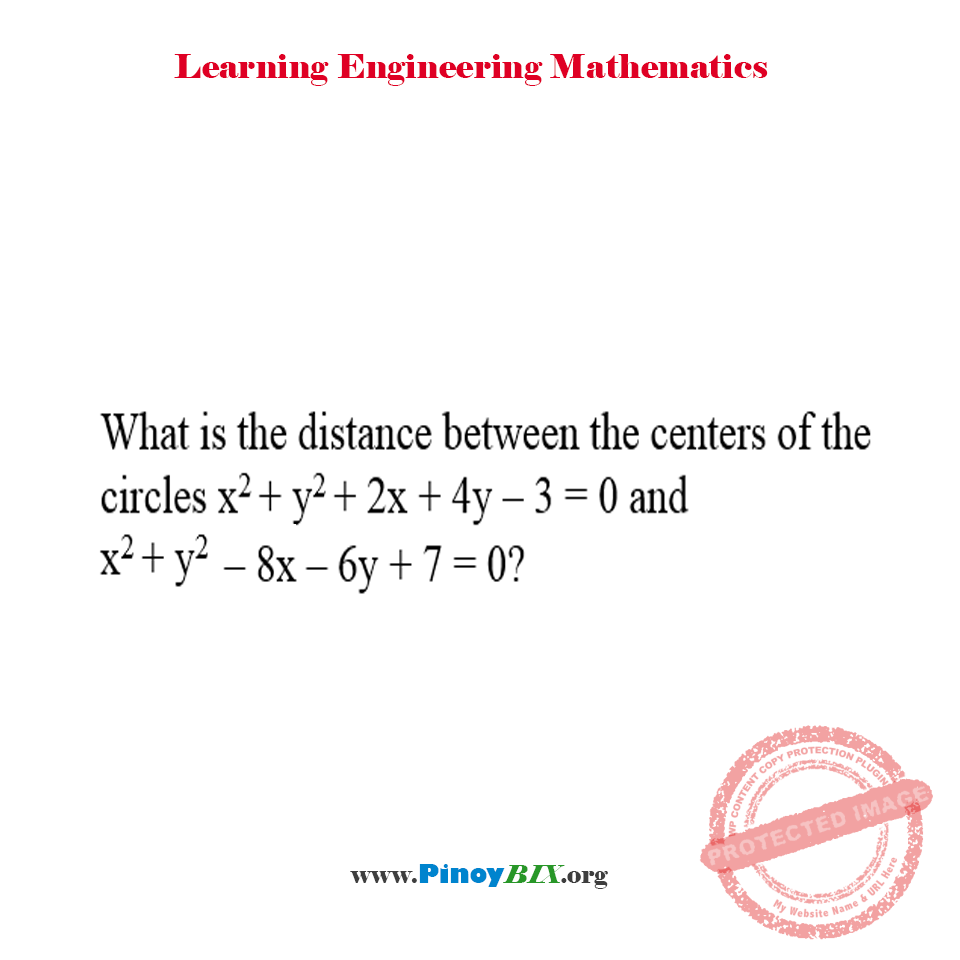Solution: What is the distance between the centers of the circles?

(Last Updated On: November 16, 2021)Problem Statement:

What is the distance between the centers of the circles x^2 + y^2 + 2x + 4y – 3 = 0 and x^2 + y^2 – 8x – 6y + 7 = 0?

The distance between the centers of the circles is equal to 7.07 units.

Latest Problem Solving in Analytic Geometry Problems (Circles, Parabola, Ellipse, Hyperbola)

More Questions in: Analytic Geometry Problems (Circles, Parabola, Ellipse, Hyperbola)

Online Questions and Answers in Analytic Geometry Problems (Circles, Parabola, Ellipse, Hyperbola)

PinoyBIX Engineering. © 2014-2021 All Rights Reserved | How to Donate? |